Computer Science

# Lab 1b — Functions

## Preparation

### 5 minutes

Create a folder for `lab1b`.

Your folder organization should look like this:## Exercise 1

### 5 minutes

Inside of `lab1b`, create a file called `exercise1.py`. Copy and paste the following code into this file.

``````from byubit import Bit

def draw_one_dot(bit):
bit.move()
bit.left()
bit.move()
bit.paint('blue')
bit.right()
bit.right()
bit.move()
bit.left()
bit.move()

@Bit.empty_world(9, 3)
def dots(bit):
draw_one_dot(bit)

if __name__ == '__main__':
dots(Bit.new_bit)
``````

Run this code, and you should see that it draws a single dot in the middle row: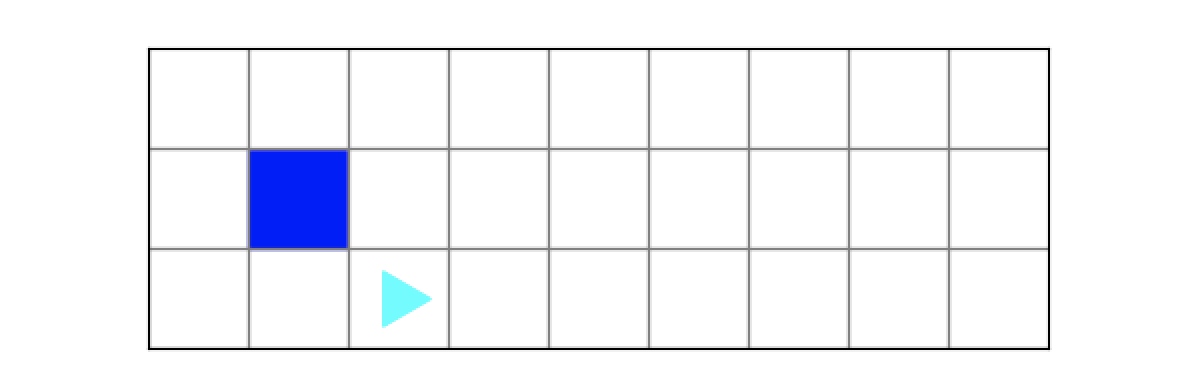Modify the `dots()` function so that it draws a total of four dots, each one separated by a blank square: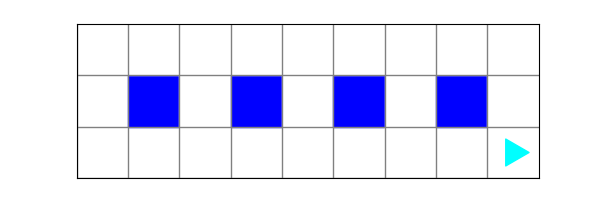## Exercise 2

### 5 minutes

Inside of `lab1b`, create a file called `exercise2.py`. Copy and paste the following code into this file.

``````from byubit import Bit

def draw_one_dot(bit):
bit.left()
bit.move()
bit.paint('blue')
bit.right()
bit.right()
bit.move()
bit.left()

@Bit.empty_world(8, 3)
def dots(bit):
draw_one_dot(bit)

if __name__ == '__main__':
dots(Bit.new_bit)
``````

Run this code, and you should see that it draws a single dot in the middle row: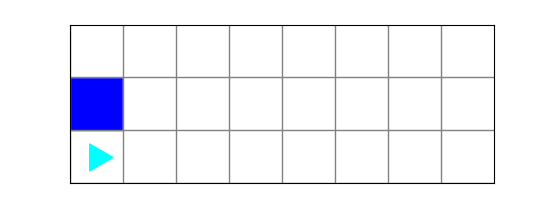Modify the `dots()` function so that it draws a total of four dots, each one separated by a blank square: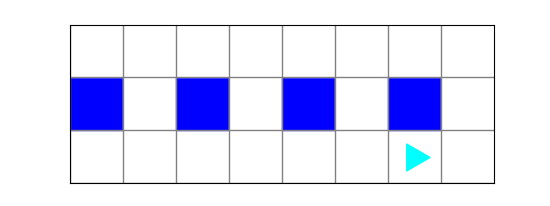You should notice that your `dots()` function requires some glue code. This is code written in between the function calls:

``````@Bit.empty_world(8, 3)
def dots(bit):
draw_one_dot(bit)
# glue code here
draw_one_dot(bit)``````

## Exercise 3

### 15 minutes

Inside of `lab1b`, create a file called `exercise3.py`. Copy and paste the following code into this file.

``````from byubit import Bit

@Bit.empty_world(5, 8)
def one_firework(bit):
pass

if __name__ == '__main__':
one_firework(Bit.new_bit)``````

When you see the keyword `pass` this is a Python command that does nothing. It is just a placeholder. Delete `pass` and write code in the `one_firework()` function.

Bit is in a 5x8 world and you want to draw one firework: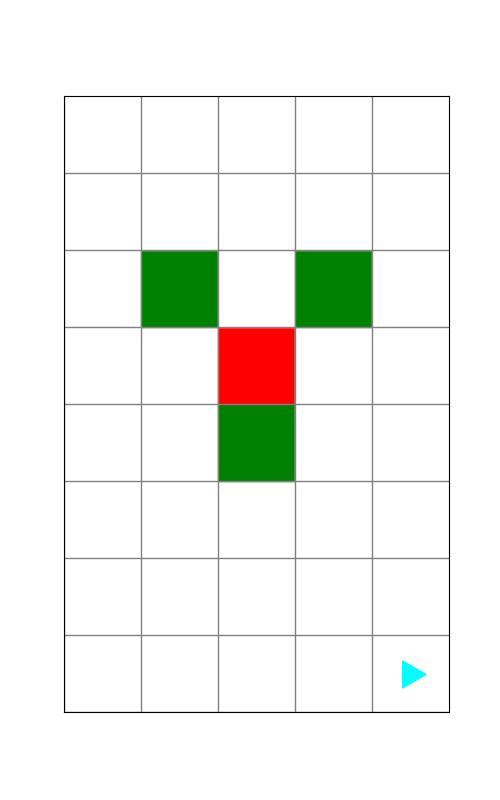You should also get Bit to be in the right position and direction after you draw the firework. Doing this will be good practice for the homework and project, where this will be enforced by the grader.

## Exercise 4

### 10 minutes

Inside of `lab1b`, create a file called `exercise4.py`. Copy and paste the following code into this file.

``````from byubit import Bit

@Bit.empty_world(17, 8)
def fireworks(bit):
pass

if __name__ == '__main__':
fireworks(Bit.new_bit)``````

Bit is now in a 17x8 world. Copy and paste your `one_firework()` function into this file, above the `fireworks()` function. Be sure to remove the `@Bit.empty_world(5, 8)` decorator from the `one_firework()` function when you copy and paste it.

Inside of `fireworks()`, write code that calls `one_firework()` to draw three fireworks:You should be able to do this without modifying your `one_firework()` function, but you will need some glue code.